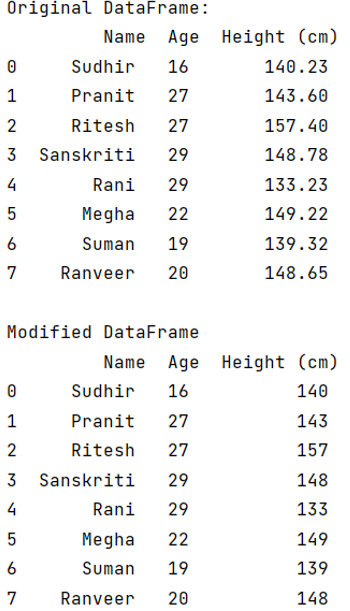# How to convert floats to ints in Pandas?

Learn how to convert floats to ints in Pandas?
Submitted by Pranit Sharma, on May 14, 2022

Pandas is a special tool which allows us to perform complex manipulations of data effectively and efficiently. Inside pandas, we mostly deal with a dataset in the form of DataFrame. DataFrames are 2-dimensional data structure in pandas. DataFrames consists of rows, columns and the data. The data inside the rows and columns can be of any type like int, float, string etc.

In this article, we are going to learn how to convert floats to ints in pandas DataFrame? For this purpose, we are going to use pandas.DataFrame.astype() method.

## pandas.DataFrame.astype()

This method is used for type casting. It cast an object to a specified dtype, it is very easy to understand when it comes to cast the data type of a particular column.

Syntax:

```DataFrame.astype(
dtype,
copy=True,
errors='raise'
)

# or
DataFrame.astype(dtype='')
```

To work with pandas, we need to import pandas package first, below is the syntax:

```import pandas as pd
```

Let us understand with the help of an example:

```# Importing pandas package
import pandas as pd

# Create a dictionary for the DataFrame
dict = {
'Name': ['Sudhir', 'Pranit', 'Ritesh','Sanskriti', 'Rani','Megha','Suman','Ranveer'],
'Age': [16, 27, 27, 29, 29,22,19,20],
'Height (cm)': [140.23, 143.6, 157.4, 148.78, 133.23,149.22,139.32,148.65]
}

# Converting Dictionary to Pandas Dataframe
df = pd.DataFrame(dict)

# Display original DataFrame
print("Original DataFrame:\n",df,"\n")

# Converting float to int
df['Height (cm)'] = df['Height (cm)'].astype(int)

# Display modified DataFrame
print("Modified DataFrame\n",df)
```

Output:Preparation